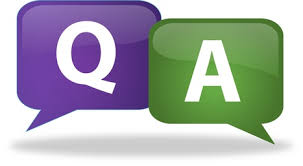# SCC Education

## CHEMICAL REACTIONS AND EQUATIONS, test paper 2CHEMICAL REACTIONS AND EQUATIONS

MARKS-30
Instructions:
 Questions : 1 to 5 – 1 Mark each
 Questions : 6 to 9 – 2 Marks each
 Questions : 10 to 13 – 3 Marks each
 Question 14 – 5 Marks

1. Burning of a candle is a chemical change; where as melting of a candle wax is a chemical change. Why?

2. What is a reducing agent?

3. What is the chemical formula of rust?

4. What is the role of catalyst in a chemical change?

5. Why does stale food give a bad smell and bad taste?

6. What are the 2 important conditions of a balanced ionic equation?

7. Why does the blue colour of Copper sulphate solution start fading when a Zinc rod is dipped in it. Write its equation.

8. Give 2 examples from everyday life situations, where redox reactions are taking place.

9. Can we place Silver nitrate in an iron vessel? Why or why not?

10. What types of reactions are represented by the following? Also give reason the same. (i)
A + B → C
(ii) A + BC → AC + B
(iii) AB + CD → AD + CB

11. What is the relationship between oxidation and oxidising agent in a redox reaction? Write an example to show this relationship.

12. What is corrosion? Why do aluminium sheets not corrode easily? Name any 2 methods to prevent corrosion.

13. Discuss the importance of decomposition reactions in metal industry.

14. (i) Give one example where corrosion is an advantage rather than disadvantage.
(ii) What is the difference between displacement reaction and double displacement reaction with suitable example?
(iii) Write 2 limitations of a chemical reaction.